Rule Detection Quiz I, Non Verbal Reasoning

# Rule Detection Quiz I, Non Verbal Reasoning - SSC CGL

Test Description

## 10 Questions MCQ Test Non Verbal Reasoning - Rule Detection Quiz I, Non Verbal Reasoning

Rule Detection Quiz I, Non Verbal Reasoning for SSC CGL 2023 is part of Non Verbal Reasoning preparation. The Rule Detection Quiz I, Non Verbal Reasoning questions and answers have been prepared according to the SSC CGL exam syllabus.The Rule Detection Quiz I, Non Verbal Reasoning MCQs are made for SSC CGL 2023 Exam. Find important definitions, questions, notes, meanings, examples, exercises, MCQs and online tests for Rule Detection Quiz I, Non Verbal Reasoning below.
Solutions of Rule Detection Quiz I, Non Verbal Reasoning questions in English are available as part of our Non Verbal Reasoning for SSC CGL & Rule Detection Quiz I, Non Verbal Reasoning solutions in Hindi for Non Verbal Reasoning course. Download more important topics, notes, lectures and mock test series for SSC CGL Exam by signing up for free. Attempt Rule Detection Quiz I, Non Verbal Reasoning | 10 questions in 20 minutes | Mock test for SSC CGL preparation | Free important questions MCQ to study Non Verbal Reasoning for SSC CGL Exam | Download free PDF with solutions
 1 Crore+ students have signed up on EduRev. Have you?
Rule Detection Quiz I, Non Verbal Reasoning - Question 1

### Directions to Solve In each of the following questions, choose the set of figures which follows the given rule. Question - Choose the set of figures which follows the given rule.Rule: Closed figures losing their sides and open figures gaining their sides.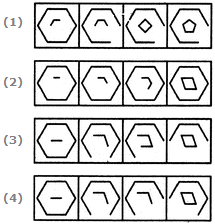Rule Detection Quiz I, Non Verbal Reasoning - Question 2

### Directions to Solve In each of the following questions, choose the set of figures which follows the given rule. Question - Choose the set of figures which follows the given rule.Rule: Closed figures gradually become open and open figures gradually become closed.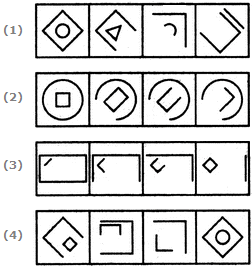Rule Detection Quiz I, Non Verbal Reasoning - Question 3

### Directions to Solve In each of the following questions, choose the set of figures which follows the given rule. Question - Choose the set of figures which follows the given rule.Rule: Any figure can be traced by a single unbroken line without retracting.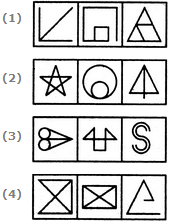Rule Detection Quiz I, Non Verbal Reasoning - Question 4

In each of the following questions, choose the set of figures which follows the given rule.

Question -

Choose the set of figures which follows the given rule.

Rule: Closed figures become more and more open and open figures become more and more closed.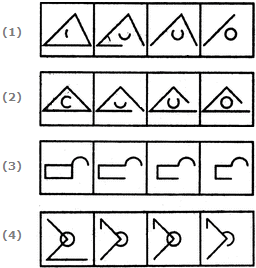Rule Detection Quiz I, Non Verbal Reasoning - Question 5

In each of the following questions, choose the set of figures which follows the given rule.

Question -

Choose the set of figures which follows the given rule.

Rule: The series becomes complex as it proceeds.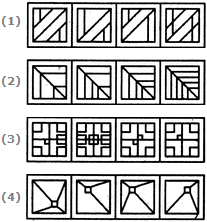Rule Detection Quiz I, Non Verbal Reasoning - Question 6

In each of the following questions, choose the set of figures which follows the given rule.

Question -

Choose the set of figures which follows the given rule.

Rule: Closed figures become more and more open and open figures become more and more closed.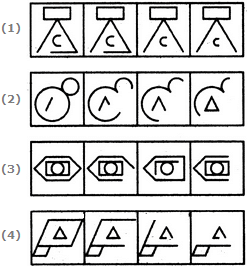Rule Detection Quiz I, Non Verbal Reasoning - Question 7

In each of the following questions, choose the set of figures which follows the given rule.

Question -

Choose the set of figures which follows the given rule.

Rule: Closed figures become more and more open and open figures become more and more closed.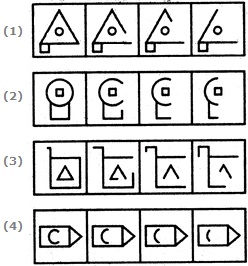Rule Detection Quiz I, Non Verbal Reasoning - Question 8

In each of the following questions, choose the set of figures which follows the given rule.

Question -

Choose the set of figures which follows the given rule.

Rule: The series becomes simpler as it proceeds.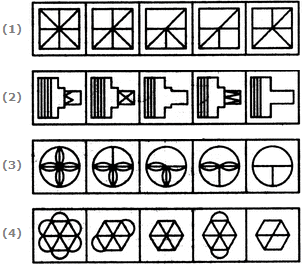Rule Detection Quiz I, Non Verbal Reasoning - Question 9

In each of the following questions, choose the set of figures which follows the given rule.

Question -

Choose the set of figures which follows the given rule.

Rule: The series becomes complex as it proceeds.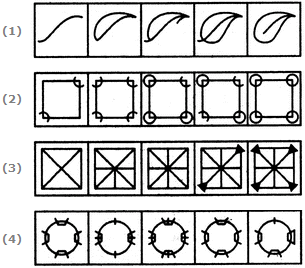Rule Detection Quiz I, Non Verbal Reasoning - Question 10

In each of the following questions, choose the set of figures which follows the given rule.

Question -

Choose the set of figures which follows the given rule.

Rule: As the circle decreases in size, its sectors increase in number.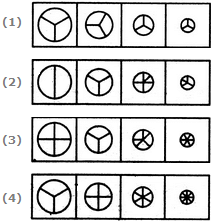## Non Verbal Reasoning

40 tests
Information about Rule Detection Quiz I, Non Verbal Reasoning Page
In this test you can find the Exam questions for Rule Detection Quiz I, Non Verbal Reasoning solved & explained in the simplest way possible. Besides giving Questions and answers for Rule Detection Quiz I, Non Verbal Reasoning, EduRev gives you an ample number of Online tests for practice

40 tests